### CONTRAST Statement

CONTRAST ’label’ contrast-specification </ options> ;

The CONTRAST statement provides a means of obtaining a test of a specified hypothesis concerning the model parameters. This is accomplished by specifying a matrixfor testing the hypothesis. You must be familiar with the details of the model parameterization that PROC GENMOD uses. For more information, see the section Parameterization Used in PROC GENMOD and the section CLASS Statement. Computed statistics are based on the asymptotic chi-square distribution of the likelihood ratio statistic, or the generalized score statistic for GEE models, with degrees of freedom determined by the number of linearly independent rows in the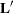matrix. You can request Wald chi-square statistics with the Wald option in the CONTRAST statement.

There is no limit to the number of CONTRAST statements that you can specify, but they must appear after the MODEL statement and after the ZEROMODEL statement for zero-inflated models. Statistics for multiple CONTRAST statements are displayed in a single table.

The elements of the CONTRAST statement are as follows:

label

identifies the contrast on the output. A label is required for every contrast specified. Labels can be up to 20 characters and must be enclosed in single quotes.

contrast-specification

identifies the effects and their coefficients from which thematrix is formed. The contrast-specification can be specified in two different ways. The first method applies to all models except the zero-inflated (ZI) distributions (zero-inflated Poisson and zero-inflated negative binomial), and the syntax is:

effect values <,…effect values>

The second method of specifying a contrast applies only to ZI models, and the syntax is:

effect values <,…effect values> @ZERO effect values <,…effect values>

where

effect

identifies an effect that appears in the MODEL statement. The value INTERCEPT or intercept can be used as an effect when an intercept is included in the model. You do not need to include all effects that are included in the MODEL statement.

values

are constants that are elements of thevector associated with the effect.

options

specifies CONTRAST statement options.

Specification of sets of effect values before the @ZERO separator results in a row of thematrix with coefficients for effects in the regression part of the model set to values and with the coefficients for the zero-inflation part of the model set to zero. Specification of sets of effect values after the @ZERO separator results in a row of thematrix with the coefficients for the regression part of the model set to zero and with the coefficients of effects in the zero-inflation part of the model set to values.

For example, the statements

class a;
model y=a;
contrast 'Label1' A 1 -1;


specify anmatrix with one row with coefficients 1 for the first level of A and –1 for the second level of A.

The statements

class a b;
model y=a / dist=zip;
zeromodel b;
contrast 'Label2' A 1 -1 @zero B 1 -1;


specify anmatrix with two rows: the first row has coefficients 1 for the first level of A, –1 for the second level of A, and zeros for all levels of B; the second row has coefficients 0 for all levels of A, 1 for the first level of B, and –1 for the second level of B.

The rows ofare specified in order and are separated by commas.

If you use the default less-than-full-rank PROC GLM CLASS variable parameterization, each row of thematrix is checked for estimability. If PROC GENMOD finds a contrast to be nonestimable, it displays missing values in corresponding rows in the results. See Searle (1971) for a discussion of estimable functions. If the elements ofare not specified for an effect that contains a specified effect, then the elements of the specified effect are distributed over the levels of the higher-order effect just as the GLM procedure does for its CONTRAST and ESTIMATE statements. For example, suppose that the model contains effects A and B and their interaction A*B. If you specify a CONTRAST statement involving A alone, thematrix contains nonzero terms for both A and A*B, since A*B contains A.

When you use any of the full-rank PARAM= CLASS variable options, all parameters are directly estimable, and rows ofare not checked for estimability.

If an effect is not specified in the CONTRAST statement, all of its coefficients in thematrix are set to 0. If too many values are specified for an effect, the extra ones are ignored. If too few values are specified, the remaining ones are set to 0.

PROC GENMOD handles missing level combinations of classification variables in the same manner as the GLM and MIXED procedures. Parameters corresponding to missing level combinations are not included in the model. This convention can affect the way in which you specify thematrix in your CONTRAST statement.

If you specify the WALD option, the test of hypothesis is based on a Wald chi-square statistic. If you omit the WALD option, the test statistic computed depends on whether an ordinary generalized linear model or a GEE-type model is specified.

For an ordinary generalized linear model, the CONTRAST statement computes the likelihood ratio statistic. This is defined to be twice the difference between the log likelihood of the model unconstrained by the contrast and the log likelihood with the model fitted under the constraint that the linear function of the parameters defined by the contrast is equal to 0. A p-value is computed based on the asymptotic chi-square distribution of the chi-square statistic.

If you specify a GEE model with the REPEATED statement, the test is based on a score statistic. The GEE model is fit under the constraint that the linear function of the parameters defined by the contrast is equal to 0. The score chi-square statistic is computed based on the generalized score function. See the section Generalized Score Statistics for more information.

The degrees of freedom is the number of linearly independent constraints implied by the CONTRAST statement—that is, the rank of.

You can specify the following options after a slash (/).

E

requests that thematrix be displayed.

SINGULAR=number
EPSILON=number

tunes the estimability checking. Ifis a vector, define ABS() to be the absolute value of the element ofwith the largest absolute value. Let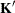be any row in the contrast matrix. Define C to be equal to ABS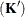if ABSis greater than 0; otherwise, C equals 1. If ABS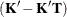is greater than C*number, then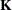is declared nonestimable.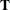is the Hermite form matrix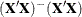, and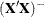represents a generalized inverse of the matrix. The value for number must be between 0 and 1; the default value is 1E–4. The SINGULAR= option in the MODEL statement affects the computation of the generalized inverse of the matrix. It might also be necessary to adjust this value for some data.

WALD

requests that a Wald chi-square statistic be computed for the contrast rather than the default likelihood ratio or score statistic. The Wald statistic for testing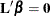is defined by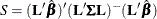where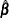is the maximum likelihood estimate and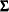is its estimated covariance matrix. The asymptotic distribution of S is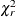, where r is the rank of. Computed p-values are based on this distribution.

If you specify a GEE model with the REPEATED statement,is the empirical covariance matrix estimate.